# 1 First Examples of Flag/Schubert Varieties (Wednesday, August 18)

Course description from Scott’s syllabus:

Schubert varieties are key examples of algebraic varieties that on one hand have an intrinsic interest and beauty, and on the other hand have many applications to algebraic geometry, algebraic topology, and representation theory; e.g., category $${\mathcal{O}}$$, infinite dimensional representation theory of real reductive groups, modular representation theory, polar varieties, Chern classes, Schubert calculus, etc.

The course goal is to understand Schubert varieties and their algebraic geometry, equivariant cohomology, and equivariant K-theory. There are many open problems related to basic geometry of Schubert varieties, so we will of course not complete this goal. One of the key applications of equivariant cohomology and equivariant K-theory of flag varieties is the complete description of the singular locus of any Schubert variety, and we will settle on learning this theory as our goal. This result was originally obtained by the author of our course textbook, and is described completely by him in Chapter XII.

The language of this result is naturally and originally described in the ominous generality of (possibly infinite dimensional) Kac-Moody groups - which are becoming increasingly more important in many areas - and the result at the time was new even for the finite dimensional case. In fact much recent literature on Schubert varieties is written in this language and at the same time is new for the finite dimensional case.

The goal of this course: describe the singular locus of arbitrary Schubert varieties. Note that we’ll assume all varieties and schemes are reduced!

References:

• Introduction to Lie Algebras and Representation Theory, Humphreys.
• Representations of Semisimple Lie Algebras in the BGG Category $${\mathcal{O}}$$, Humphreys.
• Linear Algebraic Groups, Humphreys.
• Linear Algebraic Groups, Springer.
• Kac-Moody Groups, their Flag Varieties, and their Representation Theory, Shrawan Kumar.
• Chries-Ginzburg., particularly for $${\mathsf{K}}{\hbox{-}}$$theory of abelian categories. See Youtube lectures and course notes from Geordie’s course!
• Björner and Brenti: Combinatorics of Coxeter Groups

First up, defining the words in the course title: flag varieties, equivariant cohomology, $${\mathsf{K}}{\hbox{-}}$$theory.

• Flag variety: complete homogeneous algebraic variety, i.e. with a transitive algebraic group action.
• Cohomology: it suffices to work with $$H^*_{\mathrm{sing}}(X, A; {\mathbb{R}})$$, the relative singular cohomology. See also Borel-Moore homology.
• $${\mathsf{K}}{\hbox{-}}$$theory: The study of coherent sheaves (take the Grothendieck group on the category $$\mathsf{C} = {\mathsf{Coh}}(X)$$)

For $$T \cong ({\mathbb{C}}^{\times})^n$$ a torus, define a $$T{\hbox{-}}$$space $$X$$ as a space $$X$$ with an action $$T \times X\to X$$ which is also an algebraic morphism.

Notions of equivariance will take into account this action. For cohomology, we’ll consider a space $$E\times^T X = (E\times X)/T$$ where $$T$$ acts by $$(e, x)t \mathrel{\vcenter{:}}=(et, t^{-1}x)$$. This is not a variety, but instead an Ind-variety.

For $${\mathsf{K}}{\hbox{-}}$$theory, the version we’ll work with is the following:

Let $$m: T\times T\to T$$ be the multiplication map. For $$X$$ a $$T{\hbox{-}}$$space, a sheaf $${\mathcal{F}}\in{\mathsf{Sh}}({\mathsf{{\mathcal{O}}_X}{\hbox{-}}\mathsf{Mod}})$$, $$T{\hbox{-}}$$equivariant iff

1. There is a given isomorphism of sheaves on $$T\times X$$ written $$I: a^* {\mathcal{F}}\to{\operatorname{pr}}_2 {\mathcal{F}}$$ where $${\operatorname{pr}}_2^* :T\times X\to X$$ is projection onto the second coordinate and $$a:T\times X\to X$$ is the given action map.

2. The pullbacks by $$\operatorname{id}\times a$$ and $$m\times\operatorname{id}$$ if the isomorphism $$I$$ are given by the equation \begin{align*} {\operatorname{pr}}_{23}^* I \circ (\operatorname{id}_G \times a) I = (m\times\operatorname{id}_X)^* I .\end{align*}

3. There is an isomorphism $$I_{e\times X} = \operatorname{id}$$ and $${\mathcal{F}}= a^* {\mathcal{F}}{ \left.{{}} \right|_{{e\times X}} } \xrightarrow{\sim} {\mathcal{F}}_{e\times X} = {\mathcal{F}}$$.

Note that for $$f: X\to Y$$ and $${\mathcal{F}}\in {\mathsf{Sh}}(Y)$$, then \begin{align*} f^{\star} {\mathcal{F}}= {\mathcal{O}}_X \otimes_{f^* {\mathcal{O}}_Y} f^* {\mathcal{F}} .\end{align*} For any $$T{\hbox{-}}$$space $$X$$, $${\mathcal{O}}_X$$ has a canonical $$T{\hbox{-}}$$equivariant structure given by \begin{align*} {\operatorname{pr}}_2^* {\mathcal{O}}_X \cong {\mathcal{O}}_{T\times X} \cong a^\star {\mathcal{O}}_X .\end{align*}

Take $$X\mathrel{\vcenter{:}}={\operatorname{pt}}\cong G/G$$, since any group action is transitive and we get a complete space. This is a silly but important example! We can take $$H_G^* = H_G^*({\operatorname{pt}}) \mathrel{\vcenter{:}}= H^*_{\mathrm{sing}}({\mathbf{B}}G)$$. For $$G = {\mathbb{C}}^{\times}$$, this is a polynomial ring, and for $$T = ({\mathbb{C}}^{\times})^n$$ it’s just a polynomial ring in more variables. One can then take the constant sheaf $$\underline{{\mathbb{C}}} \in {\mathsf{Sh}}(X)$$ which is $${\mathbb{C}}$$ for $$U=X$$ and $$0$$ otherwise.

$$X\mathrel{\vcenter{:}}={\mathbb{P}}^1$$ with an action by $$G\mathrel{\vcenter{:}}={\operatorname{SL}}_2({\mathbb{C}})$$: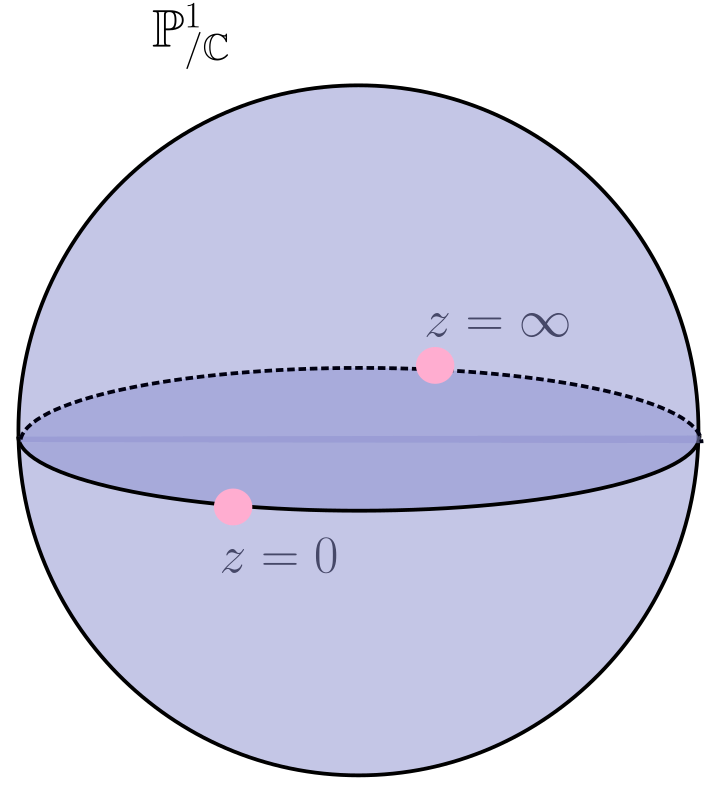In the coordinate chart $${\left[ {z_1, z_2} \right]}$$ with $$z_2\neq 0$$, we can scale $$z_2$$ to 1 and set \begin{align*} { \begin{bmatrix} {a} & {b} \\ {c} & {d} \end{bmatrix} } {\left[ {z, 1} \right]} = {\left[ {{az+b\over cz+d}, 1} \right]} && cz +d\neq 0 .\end{align*} Then

• $$G\curvearrowright X$$ transitively, and

• $$B\mathrel{\vcenter{:}}={\operatorname{Stab}}_G({\left[ {0, 1} \right]})$$ is a nontrivial Borel given by upper triangular matrices, and $$X = G/B$$.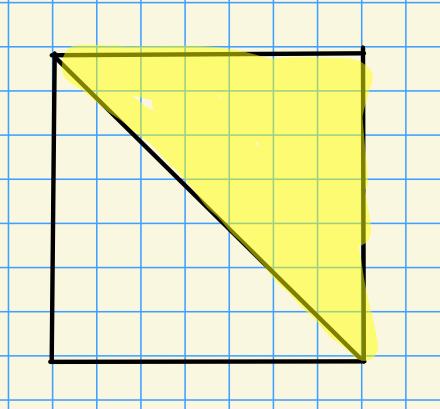Note that $${\mathcal{O}}_X({\mathbb{P}}^1) = {\mathbb{C}}$$ by Liouville’s theorem, and $${\mathcal{O}}_X(U) \cong {\mathbb{C}}[x]$$ for $$U \subseteq {\mathbb{P}}^1$$.

The Grassmannian of $$k{\hbox{-}}$$planes, given by \begin{align*} X^Y\mathrel{\vcenter{:}}={\operatorname{Gr}}_k({\mathbb{C}}^n) = \left\{{E \subseteq {\mathbb{C}}^n {~\mathrel{\Big\vert}~}\dim(E) = k}\right\} .\end{align*} This has the structure of an algebraic group, either by taking some transitive algebraic group action and lifting structure from the quotient, or taking a Segre embedding. For notation, write $${\mathbb{C}}^i \mathrel{\vcenter{:}}={\operatorname{span}}_{\mathbb{C}}\left\{{e_1,\cdots, e_i}\right\}$$ for the span of the first $$i$$ standard basis vectors.

• $$G\mathrel{\vcenter{:}}=\operatorname{GL}_n$$ acts transitively by $$g.E \mathrel{\vcenter{:}}= gE$$, for example by extending a basis from $$E$$ to $${\mathbb{C}}^n$$ and using that $$\operatorname{GL}_n$$ sends bases to bases, thus sending $$E\to E'$$ another $$k{\hbox{-}}$$plane.

• $${\operatorname{Stab}}_G({\mathbb{C}}^2)$$ are upper block-triangular matrices: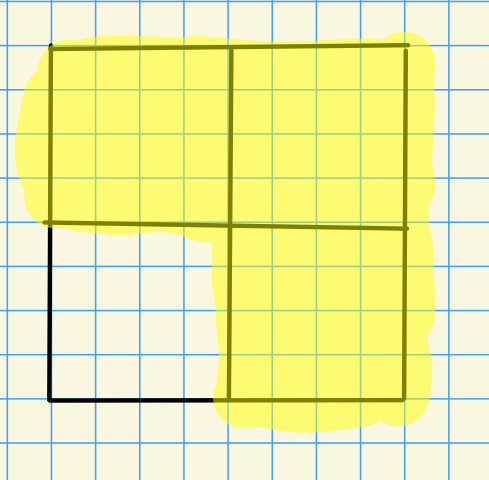Then define $$X^Y \mathrel{\vcenter{:}}= G/P$$, noting that here $$P$$ is a parabolic.

Much study of Schubert varieties reduces to studies of the combinatorics of the Weyl group. Write $$W^Y$$ for the Young diagrams on an set of $$k\times(n-k)$$ blocks.

For example, for $$n=4, k=2$$: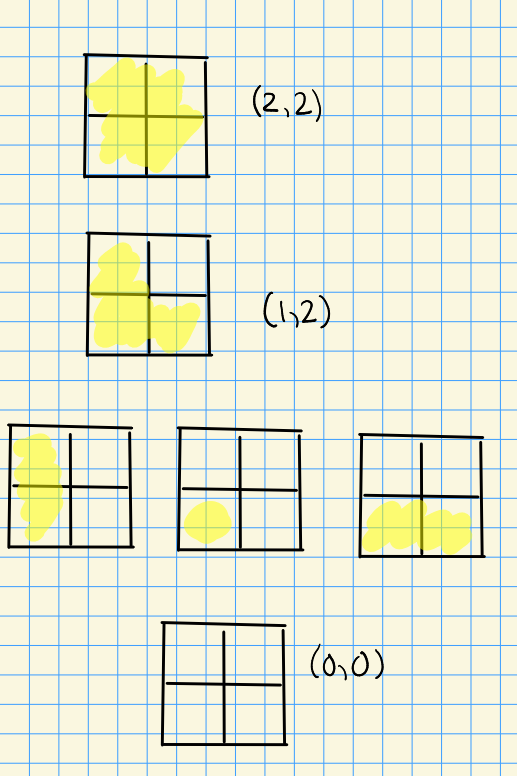For every $$\lambda\in W^Y$$, define \begin{align*} X_\lambda^Y = \left\{{E\in X^Y {~\mathrel{\Big\vert}~}\forall i=1,\cdots, k,\, \dim({\mathbb{C}}^{\sum \lambda_i + i} \cap E )\geq i}\right\} .\end{align*}

For $$\lambda = (1, 2)$$, we have \begin{align*} X_\lambda^Y = \left\{{ E \in {\operatorname{Gr}}_2({\mathbb{C}}^4) {~\mathrel{\Big\vert}~}\dim({\mathbb{C}}^2 \cap E) \geq 1, \dim({\mathbb{C}}^4 \cap E) \geq 2 }\right\} .\end{align*}

# 2 Friday, August 20

Recall that we were discussing example 3, Grassmannians, and defined $$W^Y$$ as Young diagrams in a $$k\times (n-k)$$ grid. We write \begin{align*} X^Y_{\lambda} = \left\{{ E \in X^Y = {\operatorname{Gr}}_k({\mathbb{C}}^n) {~\mathrel{\Big\vert}~}\forall 1\leq i \leq k ,\, \dim({\mathbb{C}}^{\lambda_i + i} \cap E) \geq i}\right\} .\end{align*}

\begin{align*} X_{(1, 2)}^Y = \left\{{ E {~\mathrel{\Big\vert}~}\dim({\mathbb{C}}^2 \cap E) \geq 1, \dim({\mathbb{C}}^4 \cap E)\geq 2 }\right\} .\end{align*} Note that the second condition is redundant since $$E \subset {\mathbb{C}}^4$$ is a 2-plane. Why is this a closed variety? Perhaps the easiest way to see this is using Plucker relations. Using more technology later, this allows follows from looking at $$B{\hbox{-}}$$orbits and Bruhat decompositions.

Note that for the rank function $${\operatorname{rank}}: \operatorname{Mat}(m\times n)\to {\mathbb{Z}}$$, one can compute the closure \begin{align*} \mkern 1.5mu\overline{\mkern-1.5mu\operatorname{rank}^{-1}(r)\mkern-1.5mu}\mkern 1.5mu = \operatorname{rank}^{-1}\qty{[0, r)} .\end{align*} Also note that $${\operatorname{pr}}_2: {\mathbb{C}}^r\to {\mathbb{C}}^q$$, we have $$\ker ({ \left.{{{\operatorname{pr}}_2}} \right|_{{E}} }) = {\mathbb{C}}^2 \cap E$$.

## 2.1 Example 4: The Full Flag Variety

Define the full flag variety \begin{align*} X \mathrel{\vcenter{:}}=\left\{{ F^\bullet = \qty{0 \subseteq F^1 \subseteq F^2 \subseteq \cdots \subseteq F^{n-1} \subseteq {\mathbb{C}}^n } {~\mathrel{\Big\vert}~}\dim(F^k) = k}\right\} .\end{align*}

Write $${\mathbb{C}}^\bullet \mathrel{\vcenter{:}}=\qty{0 \subseteq {\mathbb{C}}^1 \subseteq \cdots \subseteq {\mathbb{C}}^n}$$ for a distinguished basepoint.

• This is a complete homogeneous space,
• $$GL_n\curvearrowright X$$ transitively,
• $${\operatorname{Stab}}_G({\mathbb{C}}^\bullet) = B$$, the Borel of upper triangular matrices.
• $$X \cong G/B$$.

For $$G$$ a linear algebraic group and $$B$$ a closed subgroup, $$G/B$$ will generally be a variety.

The Weyl group is generally given by $$W = N_G(T)/T$$ for $$T$$ a torus.

Some facts:

• $$N_G(T)$$ is the set of permutation matrices with arbitrary nonzero entries.
• $$W = S_n$$ in general, and can be written $$W = \left\{{ (w(1), w(2), \cdots, w(n) ) {~\mathrel{\Big\vert}~}w\in S_n }\right\}$$.
• $$W \hookrightarrow X$$ sits in the flag variety via $$w\mapsto c {\mathbb{C}}^\bullet$$, i.e. acting on the distinguished basepoint.

As an example, we can write permutation matrices in one-line notation, using that $$w(e_i) = e_{w(i)}$$:

\begin{align*} A = {\left[ {e_4, e_1, e_2, e_3} \right]} \leadsto (4,1,2,3) .\end{align*}

Using that $$B/B \cong {\mathbb{C}}^\bullet$$ is the basepoint, we have $$w{\mathbb{C}}^{\bullet} = wB/B \in BwB/B$$.

\begin{align*} BwB / B \cong \left\{{ F^\bullet \in X {~\mathrel{\Big\vert}~}\forall i,j,\,\, \dim({\mathbb{C}}^i \cap F^j) \cap\dim({\mathbb{C}}^i \cap w{\mathbb{C}}^j) }\right\} .\end{align*}

Moreover, $$\dim({\mathbb{C}}^i \cap w{\mathbb{C}}^j) = \#\left\{{ k {~\mathrel{\Big\vert}~}k\leq i, w(k) \leq j}\right\}$$. Just compute $$\left\langle{e_1}\right\rangle \cap w\left\langle{e_1}\right\rangle = \left\langle{e_4}\right\rangle = 0$$ for entry $$1, 1$$, and continue: \begin{align*} \begin{bmatrix} 0 & 1 & 1 & 1 \\ 0 & 1 & 2 & 2 \\ 0 & 1 & 2 & 3 \\ 1 & 2 & 3 & 4 \\ \end{bmatrix} .\end{align*}

Now check that counting $$\left\{{k {~\mathrel{\Big\vert}~}k\leq j,\, w(k) \leq i}\right\}$$ yields the same entries in the $$i, j$$ spot, and thus the same matrix.

## 2.2 Combining Examples 3 and 4

There is a map \begin{align*} \pi: X &\to X^Y \\ F^\bullet &\mapsto F^k ,\end{align*} which is equivalently sending a Borel to its corresponding parabolic, and geometrically corresponding to sending $$T{\hbox{-}}$$fixed points to $$T{\hbox{-}}$$fixed points. This induces a map $$W\to W^Y$$, and since $$W\cong S_n$$, this is sending a Young diagram to a partition.

• This is $$G{\hbox{-}}$$equivariant for $$G\mathrel{\vcenter{:}}=\operatorname{GL}_n$$
• $$\pi(w) = \lambda$$, so there is a map $$w\to \left\{{w(1), \cdots, w(k)}\right\} = \left\{{ \lambda_1 - 1, \lambda_2 - 2, \cdots, \lambda_k - k}\right\}$$.

Given $$\lambda$$ and $$1\leq i \leq k$$, let $$w(i) = \lambda_i + i$$ and extend $$w$$ by filling in the remaining numbers in increasing order, so $$w(k+1) < w(k+2) < \cdots < w(n)$$. For example, take $$(1, 2)\mapsto w = (2, 4 {~\mathrel{\Big\vert}~}1, 3)$$, recalling that $$(1, 2)$$ has this form: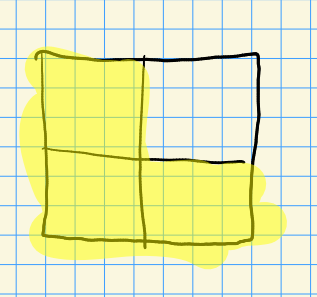One could also do $$w_{\max} = (4, 2 {~\mathrel{\Big\vert}~}3, 1)$$.

Note that the Hasse diagrams under a given diagram give the closure relations under $$B{\hbox{-}}$$orbits: For $$\lambda = (1, 2)$$, the $$B{\hbox{-}}$$orbits in $$X_\lambda^Y$$ are given by the following: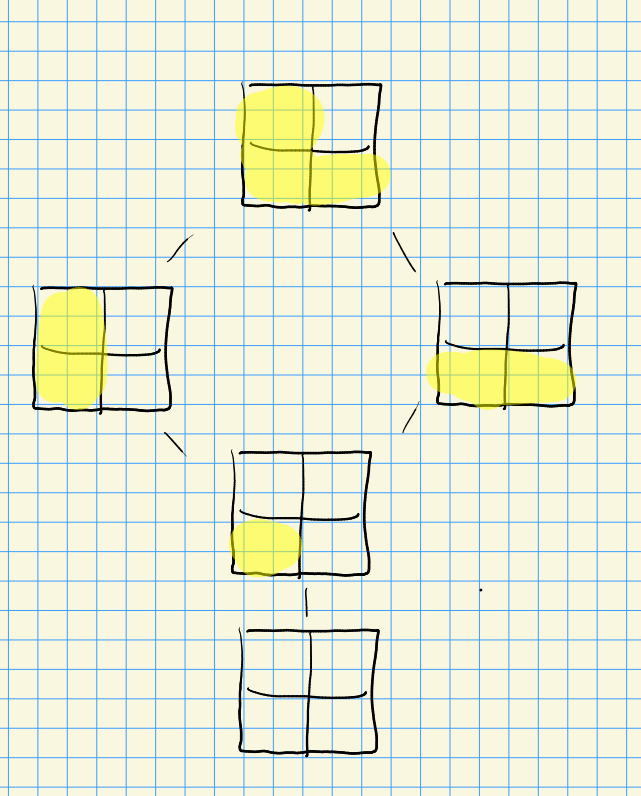We get $$BwP/P = {\mathbb{C}}^{\ell(w^r)}$$, and we in fact get a CW structure. Since $$H_{\mathrm{sing}}^2(X_\lambda^Y; {\mathbb{Z}}) \neq H_{\mathrm{sing}}^4(X_\lambda^Y; {\mathbb{Z}})$$, this doesn’t satisfying Poincare duality, so it can not be a smooth manifold. So what is the singular locus?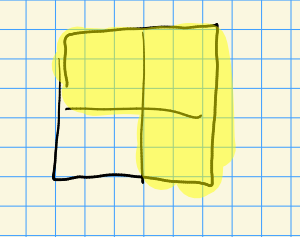One can determine that the singular locus is the single point $$\left\{{{\mathbb{C}}^2}\right\}$$ corresponding to the empty diagram: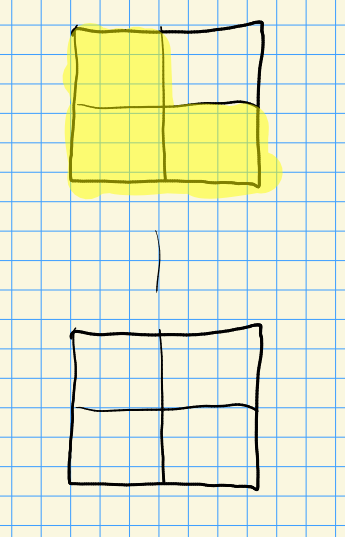# 3 Lecture 2 (Monday, August 23)

## 3.1 A Lightning Introduction to Groups and Representations

Throughout, finite type means finitely generated over the base field.

Which $$G$$ are important for equivariant cohomology of the flag variety, and equivariant $${\mathsf{K}}{\hbox{-}}$$theory. We’ll consider only connected reductive groups, and work over $$k \mathrel{\vcenter{:}}={\mathbb{C}}$$.

• A group $$G \in {\mathsf{Alg}}{\mathsf{Grp}}$$ be is a linear algebraic group if

• The coordinate ring $${\mathbb{C}}[G]$$ is a reduced (so no nonzero nilpotents) $${\mathbb{C}}{\hbox{-}}$$algebra of finite type.
• $$G$$ is a group where multiplication $$m:G^{\times 2}\to G$$ and inverseion $$i:G\to G$$ are algebraic morphisms
• A maximal torus of $$G$$ is a torus not properly contained in any other torus of the form $$({\mathbb{C}}^{\times})^{\times n}$$.

• A Cartan subgroup is the centralizer of a maximal torus. Note that maximal torii are the same as Cartans in the connected reductive case.

• $$G$$ is unipotent if every representation has a nonzero fixed vector.

• The unipotent radical $$R_u(G)\leq G$$ is a maximal closed connected normal subgroup of $$G$$.

• $$G$$ is reductive iff $$R_u(G) = \left\{{e}\right\}$$.

To study $${\mathsf{Rep}}(G)^{{\mathrm{irr}}}$$ for $$G\in {\mathsf{Alg}}{\mathsf{Grp}}$$ linear, we can assume that $$G$$ is reductive.

Let $$V\in {\mathsf{Rep}}(G)^{{\mathrm{irr}}}$$, we’ll show that the unipotent radical acts trivially. Then $$V$$ is the data of

1. $$G\to \operatorname{GL}(V)$$ for some $$V$$, a morphism of varieties and algebraic groups
2. There is an action map $$G\times V\to V$$.

Let $$V_0 = \mathrm{Fix} (R_u(G)) \subseteq V$$ be the fixed points of $$R_u(G)$$, by restricting the $$G$$ action to an $$R_u(G)\leq G$$ action by a subgroup. We know $$V_0 \neq 0$$, and we have for every $$g\in G, r\in R_u(G), v\in V_0$$. We’d like to show $$V_0 = V$$, which means that $$R_u(G)$$ acts trivially. So we’ll show $$r$$ fixes every $$gv$$: \begin{align*} r(gv) = g(g^{-1}r g)v \in g R_u(G) v = gv ,\end{align*} using that $$R_u(G)$$ fixes $$v$$. So $$V_0$$ is $$G{\hbox{-}}$$stable, and since $$V_0$$ is irreducible and $$V$$ is irreducible, we get equality.

So $$R_u(G)$$ won’t matter for irreducible representations, or in turn for equivariant $${\mathsf{K}}{\hbox{-}}$$theory, and we can assume $$R_u(G) = \left\{{e}\right\}$$ is trivial. If $$G$$ is not reductive, just replace it with $$R/R_u(G)$$, which is a reductive linear algebraic group when $$G$$ is a linear algebraic group since $$R_u(G) {~\trianglelefteq~}G$$.

Next question: how can we relate compact groups to complex reductive groups?

Let $$K \in \operatorname{Lie}{\mathsf{Grp}}$$ be compact, and set $${\mathbb{C}}[K]$$ to be the $${\mathbb{C}}{\hbox{-}}$$span of matrix coefficients of finite dimensional representations of $$K$$. For $$V$$ a finite-dimensional representation of $$K$$ (just a continuous representation of a compact group), define \begin{align*} \phi: V {}^{ \vee }\otimes_{\mathbb{C}}V &\to {\mathbb{C}}[K] \\ f\otimes v &\mapsto \qty{k \xrightarrow{\phi_{f, v}} f(kv)} .\end{align*}

$${\mathbb{C}}[K]$$ is a finite type reduced algebra. Such algebras correspond to an affine variety, i.e. it is the ring of functions on some affine variety. Thus $${\mathbb{C}}[K] = {\mathbb{C}}[G]$$ for $$G\in {\mathsf{Aff}}{\mathsf{Var}}_{/ {{\mathbb{C}}}}$$ where $$K \subseteq G$$.

1. $$G$$ is a reductive algebraic group.
2. Every locally finite continuous representation of $$K$$ extends uniquely to an algebraic representation of $$G$$, and every algebraic representation of $$G$$ restricts to a locally finite representation of $$K$$.

So despite $${\mathbb{C}}[G]$$ being infinite dimensional, every representation is contained in some finite dimensional piece. Note that there is an equivalence of categories between algebraic and compact groups, but there are differences: e.g. there are no irreducible infinite dimensional representations of compact groups.

Side note, see stuff by David Vogan!

The next result reduces representations to Cartans, which are almost tori, and is along the lines of what Langlands was originally thinking about.

There is a bijection \begin{align*} \widehat{G} \mathrel{\vcenter{:}}=\left\{{\substack{ \text{Irreducible representations} \\ \text{of }G }}\right\} &\rightleftharpoons \left\{{\substack{ \text{Irreducible dominant representations} \\ \text{of a Cartan subgroup } H\leq G }}\right\} \end{align*}

Moreover,

1. If $$G$$ is finite, $$\left\{{e}\right\} = B \supseteq = \left\{{e}\right\}$$, so there is no reduction in this case, noting that the centralizer ends up being the whole group.
2. If $$G$$ is connected reductive, then $$T=H$$ and there reduce to dominant characters of a torus.

See David Vogan’s orange book on unitary representations of real reductive groups.

Try proving this directly!

Define \begin{align*} X(T) \mathrel{\vcenter{:}}=\left\{{T \xrightarrow{f} {\mathbb{C}}^{\times}{~\mathrel{\Big\vert}~}f \text{ is algebraic}}\right\} ,\end{align*} which is a moduli of irreducible representations of $$G$$. Then \begin{align*} X(T) \supseteq D_{\mathbb{Z}}\mathrel{\vcenter{:}}=\left\{{\chi \in X {~\mathrel{\Big\vert}~}\chi \text{ is dominant for } B}\right\} .\end{align*} Note that this may make more sense after seeing root systems.

Given $$\lambda \in D_{\mathbb{Z}}$$, define a $$G{\hbox{-}}$$equivariant line bundle on the flag variety as $${\mathcal{L}}(\lambda) \mathrel{\vcenter{:}}=(G\times{\mathbb{C}}_{-\lambda})/B$$, where $$(-\lambda)t \mathrel{\vcenter{:}}=\lambda(t)^{-1}$$. This can be extended to a representation of $$B$$ by \begin{align*} B \to B/R_u(B) \cong T \xrightarrow{\lambda} {\mathbb{C}}^{\times} .\end{align*} This makes sense thinking of a Borel as upper-triangular matrices, tori as diagonal matrices, and unipotent as strictly upper triangular. So we can extend representations by making them trivial on a normal subgroup?

We refer to $$\lambda$$ as the map and $${\mathbb{C}}_{\lambda}$$ as the vector space in the representation $$G\to \operatorname{GL}(V)$$. Note that $$B$$ acts on the right of $$G\times {\mathbb{C}}_{-\lambda}$$ by \begin{align*} (g, z)b \mathrel{\vcenter{:}}=(gb, b^{-1}z) \mathrel{\vcenter{:}}=(gb, \lambda(b)^{-1}z) .\end{align*}

$${\mathcal{L}}(\lambda)$$ is an algebraic variety.

# 4 More Broad Overview (Wednesday, August 25)

We’ll assume background in affine varieties, but not necessarily sheaves. Today’s material: see Springer.

Let $$X\in {\mathsf{Top}}$$, then a ringed space is the data of $$X$$ and for all $$U\in {\mathsf{Open}}(X)$$ an assignment $${\mathcal{O}}(U) \in {\mathsf{Alg}}_{{\mathbb{C}}}$$ a $${\mathbb{C}}{\hbox{-}}$$algebra of complex functions satisfying restriction and extension, also known as a sheaf of $${\mathbb{C}}{\hbox{-}}$$valued functions. A morphism of ringed spaces $$\xi:X\to Y$$ is a continuous function such that for all $$W\in {\mathsf{Open}}(Y)$$, one can form the pullback \begin{align*} \xi_W^*f: \xi^{-1}(W) \xrightarrow{\xi} W \xrightarrow{f} {\mathbb{C}} ,\end{align*} and we require that there is a well-defined induced map $$\xi_W^*: {\mathcal{O}}_Y(W) \to {\mathcal{O}}_X(\xi^{-1}(W))$$.

For $$X$$ an affine variety, the sheaf $${\mathcal{O}}_X$$ of regular functions satisfies this property. Note that $${\mathcal{O}}$$ can be an arbitrary sheaf though, not necessarily just regular functions.

A prevariety $$X$$ is a quasicompact space $$X$$ such that every $$x\in X$$ admits a neighborhood $$U \subseteq X$$ such that $$(U, \mathop{\mathrm{Res}}({\mathcal{O}}_X, U))$$ is isomorphic to an affine variety. A prevariety is a variety if it is additionally separated, so $$\Delta_X \subseteq X^{\times 2}$$ is closed.

Last time we said that $${\mathcal{L}}(\lambda)$$ is an algebraic variety, so it satisfies the above definitions.

From now on $$G$$ will be a connected reductive group. $$\pi: G\to {\mathcal{L}}(\lambda)$$ will always be the map from the group to the flag variety.

Let $$X \in {\mathsf{Alg}}{\mathsf{Var}}_{/ {{\mathbb{C}}}}$$ and $$H\in {\mathsf{Alg}}{\mathsf{Grp}}$$ be linear where $$H\curvearrowright X$$. Then $$X/H$$ is a quotient in $${\mathsf{Top}}$$, by just taking the quotient topology. Let $$\rho: X\to X/H$$ be the projection, then define the ring of functions as \begin{align*} {\mathcal{O}}_{X/H}(U) \mathrel{\vcenter{:}}=\left\{{f\in \mathop{\mathrm{Hom}}(U, {\mathbb{C}}) {~\mathrel{\Big\vert}~}\mathop{\mathrm{Res}}(f \circ \rho, \rho^{-1}(U) ) \in {\mathcal{O}}_X(\rho^{-1}(U))}\right\} .\end{align*} In this way $${\mathcal{O}}_{X/H}(U)$$ can be identified with $$H{\hbox{-}}$$invariant functions $${\mathcal{O}}_X(\rho^{-1}(U))^H$$. This makes $$X/H$$ a ringed space, which is often (but not necessarily) an algebraic variety.

This is not always an algebraic variety, e.g. taking $${\mathbb{C}}^{\times}\curvearrowright{\mathbb{C}}$$ by multiplication. This yields two orbits (0 and everything else) and isn’t a variety.

If $$\pi: G\to G/H$$ has local sections, then $$(G\times X)/H \in {\mathsf{Alg}}{\mathsf{Var}}$$ using $$(g, x)h \mathrel{\vcenter{:}}=(gh, h^{-1}x)$$. Note that this is a fiber bundle for the Zariski topology, and doesn’t have local sections (contrasting the analytic topology).

The map $$\pi: G\to G/B$$ has local sections (but no global sections).

Side note: we have the Bruhat decomposition $$G = {\textstyle\coprod}_{w\in W} BwB$$ as a partition into double cosets, quotienting by an action of $$B\times B$$. The theorem is that these are parameterized by the Weyl group.

Let $$B = TU$$ where $$T$$ is a torus and $$U$$ unipotent (so upper triangular, ones along the diagonal) and set $$U^-$$ to be the opposite unipotent radical (e.g. lower triangular, ones along diagonal). Define a map \begin{align*} \phi: U^i \times B &\to G \\ (\mkern 1.5mu\overline{\mkern-1.5muu\mkern-1.5mu}\mkern 1.5mu, b) &\mapsto \mkern 1.5mu\overline{\mkern-1.5muu\mkern-1.5mu}\mkern 1.5mub^{-1} .\end{align*} Then $$\operatorname{im}(\phi) = U^- B$$, and $$\phi$$ is injective since $$U^- \cap B = \left\{{e}\right\}$$. The argument on matrices holds more generally: $$B$$ are the upper triangular matrices and $$U^-$$ has ones on the diagonal, so these intersect only at the identity. $$\phi$$ is an open embedding: one can show that the derivative is surjective: \begin{align*} d\phi(1, 1): {\mathfrak{u}}^- \times {\mathfrak{b}}&\to {\mathfrak{y}}\\ (x, y) &\mapsto x-y .\end{align*} Rewriting the target as $${\mathfrak{u}}^- \oplus {\mathfrak{h}}\oplus {\mathfrak{u}}^+$$ where $${\mathfrak{b}}= {\mathfrak{h}}\oplus {\mathfrak{u}}^+$$, one can find preimages of any element.

Define a local section: $$\sigma: U\to G$$ where $$U \subseteq G/B$$. Use the composite $$U^- \times B\to G \to G/B \supseteq U^-$$ to view $$U^-$$ as a subset of the flag variety. An explicit formula for section is the following: \begin{align*} \sigma(\mkern 1.5mu\overline{\mkern-1.5muu\mkern-1.5mu}\mkern 1.5mu) \mathrel{\vcenter{:}}=(\mkern 1.5mu\overline{\mkern-1.5muu\mkern-1.5mu}\mkern 1.5mu, 1) \in U^- \times B \subseteq G .\end{align*} Although this only constructs a section for one open set, translating by elements of $$g$$ yields an open cover, and everything is equivariant.

Using this, $$(G\times X)/B$$ is always an algebraic variety, since $$G\to G/B$$ always has local sections. For other groups, $$X$$ quasiprojective will also make the quotient algebraic, but the proof is more difficult. However it still involves constructing local sections. It turns out that $$G{ \underset{\scriptscriptstyle {B} }{\times} }X\to G/B$$ is a locally trivial fiber bundle.

A note on notation: $$(G\times X)B$$ is sometimes written $$G{ \underset{\scriptscriptstyle {B} }{\times} } X$$ (as above), but this is not a fiber product. In this notation, $${\mathcal{L}}(\lambda) = G{ \underset{\scriptscriptstyle {B} }{\times} } {\mathbb{C}}_{- \lambda}$$. Note that this is a line bundle on $$G/B$$, so we can take sections.

1. There is a correspondence \begin{align*} H^0(G/B; {\mathcal{L}}(\lambda)) \cong \left\{{f:G\to {\mathbb{C}}{~\mathrel{\Big\vert}~}f(g) = bf(gb) }\right\} && G \in {\mathbb{C}}[G] .\end{align*}

A section $$\sigma: G/B \to G{ \underset{\scriptscriptstyle {B} }{\times} } {\mathbb{C}}_{- \lambda}$$ gets sent to $$\sigma(gB/B) = [g, f(g)]$$. Use that the quotient acts like a tensor over $$B$$, so \begin{align*} gB/B = gbB/B = [gb, f(gb)] = [g, b f(gb)] .\end{align*}

1. $$H^0(G/B; {\mathcal{L}}(\lambda)) = L_{\lambda} {}^{ \vee }$$ for $$\lambda$$ a dominant character in $$D_{\mathbb{Z}}$$, where $$L_\lambda$$ is the irreducible finite dimensional representation of $$G$$ with highest weight $$\lambda$$. Note that in the finite case, we have $$L_\lambda {}^{ \vee }= L_{w_0 \lambda}$$, but in the Kac-Moody case one doesn’t have $$w_0$$.

For $$\lambda = 0 \in X(T)$$ a character, we get \begin{align*} \left\{{f:G\to {\mathbb{C}}{~\mathrel{\Big\vert}~}f(g) = f(gb)}\right\} = {\mathbb{C}}[G/B] = {\mathcal{O}}_{G/B}(G/B)={\mathbb{C}} .\end{align*}

Chapter 1 of Kumar, Cartan matrices.

# 5 Starting Kumar (Friday, August 27)

## 5.1 1.1: Definition of Kac-Moody Algebras

Let $$A\in \operatorname{Mat}(\ell\times\ell, {\mathbb{C}})$$ be rank $$r$$. A realization of $$A$$ is a triple $$({\mathfrak{h}}, \pi, \pi {}^{ \vee })$$ where $$h\in{\mathsf{{\mathbb{C}}}{\hbox{-}}\mathsf{Mod}}$$, $$\pi = \left\{{\alpha_1, \cdots, \alpha_\ell}\right\} \subseteq {\mathfrak{h}} {}^{ \vee }$$ are column vectors, and $$\left\{{ \alpha_1 {}^{ \vee }, \cdots, \alpha_\ell {}^{ \vee }}\right\} \subseteq {\mathfrak{h}}$$ are row vectors are indexed sets satisfying

1. $$\pi, \pi {}^{ \vee }$$ are linearly independent sets.
2. $$\alpha_j( \alpha_i {}^{ \vee }) = a_{i, j}$$
3. $$\ell - r = \dim_{\mathbb{C}}({\mathfrak{h}}) - \ell$$

There exists a realization of $$A$$ that is unique up to isomorphism. Moreover, realizations of $$A, B$$ are isomorphic iff $$B$$ is similar to $$A$$ via a permutation of the index set.

Assume $$A$$ is of the form \begin{align*} A = \begin{bmatrix} A_1 \\ A_2 \end{bmatrix} ,\end{align*} where $$A_1$$ is $$r\times \ell$$ block where $$\operatorname{rank}A_1 = r$$ and $$A_2$$ is $$l-r\times \ell$$ Set \begin{align*} C \mathrel{\vcenter{:}}= \begin{bmatrix} A_1 & 0 \\ A_2 & I_{\ell-r} \end{bmatrix}\in \operatorname{Mat}(\ell \times (2\ell - r)) .\end{align*} For $${\mathfrak{h}}= {\mathbb{C}}^{2\ell - r}$$, set $$\alpha_1, \cdots, \alpha_\ell$$ to be the first $$\ell$$ coordinate functions $$\alpha_1 {}^{ \vee },\cdots$$ as the rows of $$C$$. This is a realization.

Conversely, given a realization $$({\mathfrak{h}}, \pi, \pi {}^{ \vee })$$, we can produce a matrix: complete $$\pi$$ to a basis of $${\mathfrak{h}} {}^{ \vee }$$. This can done in such a way that $$\alpha_j(\alpha_i {}^{ \vee }) = [A_1, B; A_2, D^{-1}]\in \operatorname{Mat}(\ell \times 2\ell -r$$. Using column operations, i.e. multiplication on the right, this can be mapped to $$[A_1, 0; A_2, I]$$.

Let $$V\in {\mathsf{{\mathbb{C}}}{\hbox{-}}\mathsf{Mod}}$$ and $$T^\bullet(V)$$ be its (associative) tensor algebra. Set $$[ab] = ab-ba$$ and take $$F(V) \subseteq T(V)$$ to be the free Lie algebra generated by $$T^1(V)$$. We call $$F(V)$$ the free Lie algebra generated by $$V$$. There is a universal property: for any linear hom $$\theta: V\to {\mathfrak{sl}}$$, there is a commuting diagramNote that $$U(F(V)) = T(V)$$.

This can be constructed as \begin{align*} {\mathfrak{h}}\oplus \left\langle{e_1, \cdots, e_\ell}\right\rangle \bigoplus \left\langle{f_1, \cdots, f_\ell}\right\rangle / \sim \\ \\ \sim \mathrel{\vcenter{:}}= \begin{cases} [e_i f_i] = \delta_{ij} \alpha_i {}^{ \vee }& i, j = 1,\cdots, \ell \\ [hh'] = 0 & h,h'\in{\mathfrak{h}} \\ [he_i = \alpha_i(h)e_i & \\ [hf_i = \alpha_i(h)f_i & i=1,\cdots,\ell, h\in {\mathfrak{h}} \end{cases} .\end{align*} Then set $$\tilde {\mathfrak{g}}(A) \mathrel{\vcenter{:}}= F(V)/\sim$$ We’ll find that this only depends on the realization of $$A$$.

A matrix $$A = (\alpha_{ij})$$ is a generalized Cartan matrix (GCM):

• $$\alpha_{ii} = 2$$
• $$\alpha_{ij} \leq 0, i\neq j$$
• $$\alpha_{ij} = 0$$ if $$\alpha_{ji} =0$$

The Kac-Moody Lie algebra is defined by $${\mathfrak{g}}\mathrel{\vcenter{:}}={\mathfrak{g}}(A) \mathrel{\vcenter{:}}=\tilde{\mathfrak{g}}(A)/\sim$$, where we mod out by the Serre relations: \begin{align*} (\operatorname{ad}e_i)^{1 - a_{ij}}(e_j) &= 0\\ (\operatorname{ad}f_i)^{1 - a_{ij}}(f_j) &= 0 .\end{align*}

• There is an injection $${\mathfrak{h}}\hookrightarrow{\mathfrak{g}}$$, so we refer to $${\mathfrak{h}}$$ as the Cartan subalgebra.
• The $$e_i, f_i$$ are Chevalley generators.
• The nilradicals are $${\mathfrak{n}}\mathrel{\vcenter{:}}=\left\langle{\left\{{e_1,\cdots,e_\ell}\right\}}\right\rangle$$ and $${\mathfrak{n}}^- \mathrel{\vcenter{:}}=\left\langle{ \left\{{f_1, \cdots, f_\ell}\right\}}\right\rangle$$.
• $${\mathfrak{b}}\mathrel{\vcenter{:}}={\mathfrak{h}}\oplus {\mathfrak{n}}$$ is the standard Borel.
• $${\mathfrak{b}}^- \mathrel{\vcenter{:}}={\mathfrak{h}}\oplus {\mathfrak{n}}$$
• $$\tilde {\mathfrak{n}}, \tilde {\mathfrak{n}}^-, \tilde {\mathfrak{b}}, \tilde {\mathfrak{b}}^-$$ can similarly be defined for $$\tilde {\mathfrak{g}}$$.

A big theorem from algebraic groups: a connected reductive group $$G$$ corresponds to a root datum$$({\mathfrak{g}}, \left\{{\alpha_i}\right\}_{i\leq \ell}, \left\{{ \alpha_i {}^{ \vee }}\right\}_{i\leq \ell} )$$ where $$\alpha_i, \alpha_i {}^{ \vee }\in {\mathbb{Z}}^n$$ such that $$a_{ij} \mathrel{\vcenter{:}}={\left\langle {\alpha_i},~{\alpha_i {}^{ \vee }} \right\rangle}$$ form a Cartan matrix $$A \mathrel{\vcenter{:}}=(a_{ij})$$.

Consider pairs of $$K, G$$ where $$G$$ is the complexification of $$K$$:

• $${\mathsf{Sp}}_n \leadsto {\mathsf{Sp}}_{2n}({\mathbb{C}})$$, $$Z(G) = {\mathbb{Z}}/2$$ for $$n\geq 1$$
• $${\operatorname{SU}}_n \leadsto {\operatorname{SL}}_n({\mathbb{C}})$$, $$Z(G) = {\mathbb{Z}}/4n$$ for $$n\geq 3$$
• $${\operatorname{Spin}}_n \leadsto {\operatorname{Spin}}_n({\mathbb{C}})$$, $$Z(G) = ({\mathbb{Z}}/2)^2$$, $$n\geq 8$$ even
• $$F_4$$, $$Z(G) = {\mathbb{Z}}/4$$ for $$n\geq 7$$ odd
• $$G_2$$
• $$E_6$$
• $$E_7$$
• $$E_8$$

Here we take the simply connected groups for the last 5, and the last 4 have cyclic centers.

There exist

1. Simple, simply connected, connected groups $$G_1, \cdots, G_k$$,
2. A finite central subgroup $$F \subseteq \prod G_i \times T'$$ where $$T'$$ is a (not necessarily maximal) torus,

such that $$G\cong (\prod G_i \times T')/F$$. All connected reductive groups arise this way!

Let $$G\mathrel{\vcenter{:}}=\operatorname{GL}_n = {\operatorname{SL}}_n \cdot {\mathbb{C}}^{\times}$$, and they intersect at roots of unity, so \begin{align*} \operatorname{GL}_n = ({\operatorname{SL}}_n \times {\mathbb{C}}^{\times}) / \left\langle{\zeta_n I_n, \zeta_n^{-1}}\right\rangle .\end{align*} The map (in the reverse direction) is $$(g, z)\mapsto gz$$, and if $$gz= I$$ in $$\operatorname{GL}_n$$ then $$g = \zeta_n^k I_n$$ and $$z = \zeta_k^{-1}$$.

Assume $$G$$ is semisimple, simply connected, and connected. Then

1. The equivariant cohomology is \begin{align*} H^*_T(G/B; {\mathbb{Q}}) \cong S_{\mathbb{Q}}\otimes_{S_{\mathbb{Q}}^W} S_{\mathbb{Q}} \end{align*}
2. The equivariant $${\mathsf{K}}{\hbox{-}}$$theory \begin{align*} K^T(G/B) = A(T) \otimes_{A(T)^W}A(T) \end{align*}

Note that \begin{align*} W &= N_G(T)/T \\ S &= S({\mathfrak{h}} {}^{ \vee }), && \pi \subseteq {\mathfrak{h}} {}^{ \vee }\\ A(T) &= {\mathbb{Z}}[X(T)] .\end{align*}

Think about semisimple, simply connected, and connected groups most of the semester.

# 6 Kac-Moody Groups (Monday, August 30)

See exercises in first two sections, 1.1 and 1.2. See also the proof of the Borel-Weil theorem.

## 6.1 1.2: Root Space Decompositions

Starting with a generalized Cartan matrix $$A$$, we produced a Lie algebra $$\tilde{\mathfrak{g}}(A)$$ by taking the free Lie algebra and modding out by certain relations. This algebra only depended on the realization of $$A$$, namely $$({\mathfrak{g}}, \pi, \pi {}^{ \vee })$$, which we thought of as $$({\mathfrak{g}},{\mathfrak{h}} {}^{ \vee }, {\mathfrak{h}})$$, yielding $${\mathfrak{g}}(A)$$ modulo Serre relations.

Define \begin{align*} Q &\mathrel{\vcenter{:}}={\mathbb{Z}}\pi \subseteq {\mathfrak{h}} {}^{ \vee }&& \text{the root lattice }\\ Q^+ &\mathrel{\vcenter{:}}={\mathbb{Z}}_{\geq 0} \pi \subseteq {\mathfrak{h}} {}^{ \vee }\\ {\mathfrak{g}}_\alpha &\mathrel{\vcenter{:}}=\left\{{x\in g {~\mathrel{\Big\vert}~}\forall h\in{\mathfrak{h}}, [hx] = \alpha(h) x}\right\} && \text{for }\alpha\in{\mathfrak{h}} {}^{ \vee } .\end{align*}

1. $${\mathfrak{g}}= {\mathfrak{n}}^- \oplus {\mathfrak{h}}\oplus {\mathfrak{n}}$$, which are all nonzero.
2. $${\mathfrak{n}}^{\pm \alpha} = \bigoplus_{\alpha\in Q^+\setminus\left\{{{\operatorname{pt}}}\right\}} {\mathfrak{g}}_{\pm \alpha}$$.
3. $$\dim_{\mathbb{C}}{\mathfrak{g}}_\alpha < \infty$$.
4. $${\mathfrak{n}}\mathrel{\vcenter{:}}=\left\langle{e_1,\cdots, e_\ell}\right\rangle$$ subject only to the Serre relations, i.e. no additional relations are needed for this subalgebra.

First step: prove for $$\tilde {\mathfrak{g}}$$ and put a tilde on everything appearing in the theorem statement.

Let $$\left\{{v_1, \cdots, v_\ell}\right\}$$ be a basis for $$V$$ and fix $$\lambda \in {\mathfrak{h}} {}^{ \vee }$$. Define an action of generators of $$\tilde {\mathfrak{g}}$$ on $$T(V)$$ in the following way:

1. $$\alpha:$$ Set $$f_i(\alpha) \mathrel{\vcenter{:}}= v_i\otimes a$$ for $$a\in T(V)$$
2. $$\beta:$$ set $$h(1) \mathrel{\vcenter{:}}={\left\langle {\lambda},~{h} \right\rangle}1 \mathrel{\vcenter{:}}=\lambda(h)\cdot 1$$, and inductively on $$s$$ set \begin{align*} h(v_j \otimes a) \mathrel{\vcenter{:}}=- {\left\langle {\alpha_j},~{h} \right\rangle} v_j \otimes a + v_j \otimes h(a) && a\in T^{s-1}(V), h\in {\mathfrak{h}}, 1\leq j \leq \ell .\end{align*}
3. $$\gamma:$$ Set $$e_i(1) \mathrel{\vcenter{:}}= 0$$ to kill constants, and inductively on $$s$$, \begin{align*} e_i(v_j \otimes a) = \delta_{ij}\alpha {}^{ \vee }_{i}(a) + v_j\otimes e_i(a) && a\in T^{s-1}(V), 1\leq j \leq \ell .\end{align*}

One should show that these define a representation by checking the Serre relations. Consider instead how this works in the $${\mathfrak{g}}= {\mathfrak{sl}}_2$$ case:

For $${\mathfrak{sl}}_2$$, take the realization $$({\mathbb{C}}, \left\{{\alpha}\right\}, \left\{{ \alpha {}^{ \vee }}\right\} )$$ corresponds to the matrix $$A = (s)$$. Here $$T(V) = {\mathbb{C}}[x]$$, and since there are no Serre relations, $$\tilde {\mathfrak{g}}= {\mathfrak{g}}$$. We have $$e = [0,1; 0,0], f = [0,0; 1,0]$$ which generate the positive/negative unipotent parts respectively. Then $$h = \left\{{ \operatorname{diag}(h, -h) }\right\}$$. Checking the action:

1. $$\alpha: { \begin{bmatrix} {0} & {0} \\ {1} & {0} \end{bmatrix} } p = xp$$ which raises degree by 1. M

2. \begin{align*} \beta: h(1) = \lambda(h)1 \implies h(xp) = -\alpha(h)xp + x(hp) ,\end{align*} where $$p\in {\mathbb{C}}[x]_{g-1}$$. For example, \begin{align*} h(x) &= -\alpha(x) + x \lambda(h) = (\lambda - \alpha)(h)x \\ h(x^2) &= (\lambda - 2\alpha)(h)x^2 ,\end{align*} so this acts diagonally and preserves degree.

3. Check \begin{align*} { \begin{bmatrix} {0} & {1} \\ {0} & {0} \end{bmatrix} } \cdot (1) &= 0 \\ { \begin{bmatrix} {0} & {1} \\ {0} & {0} \end{bmatrix} } \cdot (xp) &= p + x { \begin{bmatrix} {0} & {1} \\ {0} & {0} \end{bmatrix} } p .\end{align*}

Check that $$ex = 1+0$$ and $$ex^2 = x+x = 2x$$, so $$e$$ acts by differentiation.

Note that $${\mathfrak{h}}$$ forms a subalgebra since it’s a nondegenerate map. This follows from the fact that we get a representation $$\rho_{\lambda}$$ of $$\tilde{\mathfrak{g}}$$ on $$T(V)$$, which for each $$h$$ acts nontrivially on some $$T(V)$$. So use $$\rho_{\lambda}$$ to deduce the theorem for $$\tilde{\mathfrak{g}}$$: \begin{align*} \left\{{ [ x, y] {~\mathrel{\Big\vert}~}x,y\in {\mathfrak{h}}= \left\langle{\left\{{e_i, f_i}\right\}_{i=1}^\ell}\right\rangle }\right\} \subseteq \tilde {\mathfrak{n}}^- + {\mathfrak{h}}+ \tilde{\mathfrak{n}} = {\mathfrak{g}} ,\end{align*} we’ll show this sum is direct.

Let $$u = n^- + h + n^+ = 0$$, then in $$T(V)$$ we have $$u(1) = n^-(1) + {\left\langle {\lambda},~{h} \right\rangle}1$$, which forces $${\left\langle {\lambda},~{h} \right\rangle} = 0$$ for all $$\lambda\in{\mathfrak{h}} {}^{ \vee }$$ and thus $$h = 0$$. Use the restriction $$\tilde{\mathfrak{g}}\to \tilde{\mathfrak{n}}$$ to get a map $$U(\tilde{\mathfrak{n}}^-)\to T(V)$$ out of the enveloping algebra, using that $$T(V)$$ is an associative algebra. Using $$f_i \mapsto v_i$$, this is surjective and in fact an isomorphism. Sending $${\mathfrak{n}}^- \mapsto {\mathfrak{n}}^-(1)$$ yields $$\tilde{\mathfrak{n}}^- \subseteq U(\tilde{\mathfrak{n}}^-) = T(V)$$. This yields $$n^- = 0$$, making the sum direct.

We can write $$\tilde{\mathfrak{n}}^- = F\left\langle{f_1,\cdots, f_\ell}\right\rangle$$
and $$\tilde{\mathfrak{n}}= F\left\langle{e_1,\cdots, e_\ell}\right\rangle$$ and by the PBW theorem, $$\dim \tilde{\mathfrak{g}}_\alpha < \infty$$. This uses that the weight spaces for $$\tilde{\mathfrak{n}}^-$$ are contained in $$U(\tilde{\mathfrak{n}}^-)$$. Note that there is a Cartan involution \begin{align*} \tilde\omega: \tilde{\mathfrak{g}}&{\circlearrowleft}\\ e_i &\mapsto -f_i \\ f_i &\mapsto -e_i \\ h &\mapsto -h .\end{align*}

Now to prove the theorem for $${\mathfrak{g}}$$ itself, write $$\tilde {\mathfrak{r}}\mathrel{\vcenter{:}}=\ker( \tilde{\mathfrak{g}}\xrightarrow{\gamma} {\mathfrak{g}}_\alpha) {~\trianglelefteq~}\tilde{\mathfrak{g}}$$. This is an ideal, and thus $${\mathfrak{h}}{\hbox{-}}$$stable. We can thus write \begin{align*} \tilde{\mathfrak{r}}= \qty{ \bigoplus_{\alpha\in Q^+ \setminus\left\{{{\operatorname{pt}}}\right\}} {\mathfrak{r}}_{-\alpha} } \oplus \tilde{\mathfrak{r}}_0 \oplus \qty{ \bigoplus_{\alpha\in Q^+ \setminus\left\{{{\operatorname{pt}}}\right\}} {\mathfrak{r}}_{\alpha} } \end{align*} where $$\tilde{\mathfrak{r}}_{\beta} \mathrel{\vcenter{:}}=\tilde{\mathfrak{r}}\cap\tilde{\mathfrak{g}}_{\beta}$$ and $$\tilde {\mathfrak{r}}_0 = \tilde{\mathfrak{r}}\cap{\mathfrak{h}}$$. We have ideals $$\tilde{\mathfrak{r}}^{\pm} {~\trianglelefteq~}\tilde{\mathfrak{n}}^{\pm}$$, which are respectively generated by \begin{align*} \left\{{e_{i, j} = (\operatorname{ad}e_i)^{1 - a_{i, j}} (e_{j}) {~\mathrel{\Big\vert}~}i\neq j }\right\} && \left\{{f_{i, j} = (\operatorname{ad}f_i)^{1 - a_{i, j}} (f_{j}) {~\mathrel{\Big\vert}~}i\neq j }\right\} ,\end{align*} where $$\operatorname{ad}f_k (e_{i, j}) = 0$$ for all $$k$$ and $$i\neq j$$. Skipping a few things that are spelled out in the book, e.g. that $$\tilde {\mathfrak{r}}_0 = 0$$, we conclude that $$\tilde{\mathfrak{r}}= \tilde{\mathfrak{r}}^+ \oplus \tilde{\mathfrak{r}}^-$$, both of which are ideals in $$\tilde{\mathfrak{g}}$$. Since $$\tilde{\mathfrak{r}}_0 = 0$$, we get $${\mathfrak{h}}\subseteq {\mathfrak{g}}$$, and using that $$\gamma$$ is surjective we have an isomorphism of $${\mathbb{C}}{\hbox{-}}$$modules \begin{align*} {\mathfrak{g}}= \tilde{\mathfrak{g}}/\tilde{\mathfrak{r}}= \tilde{\mathfrak{n}}^-/ \tilde{\mathfrak{r}}^- \oplus {\mathfrak{h}}\oplus \tilde{\mathfrak{n}}/ \tilde{\mathfrak{r}}^+ .\end{align*}

Write $$\Delta \mathrel{\vcenter{:}}=\left\{{\alpha\in Q\setminus\left\{{{\operatorname{pt}}}\right\} {~\mathrel{\Big\vert}~}{\mathfrak{g}}_\alpha \neq 0}\right\}$$ the set of roots and $${\mathfrak{g}}_{\alpha}$$ the root space, then set \begin{align*} \Delta^+ &\mathrel{\vcenter{:}}=\Delta \cap Q^+ \\ \Delta^- &\mathrel{\vcenter{:}}=\Delta \cap(-Q^+) \\ \Delta &\mathrel{\vcenter{:}}=\Delta^+ \cup\Delta^- .\end{align*} Also for $$Y \subseteq \left\{{1,\cdots,\ell}\right\}$$ write \begin{align*} \Delta_Y &\mathrel{\vcenter{:}}=\Delta \cap\qty{\bigoplus_{i\in Y} {\mathbb{Z}}\alpha_i } \\ {\mathfrak{g}}_Y &\mathrel{\vcenter{:}}={\mathfrak{h}}\oplus \qty{ \bigoplus_{\alpha\in \Delta_Y} {\mathfrak{g}}_\alpha } .\end{align*} We say $$Y$$ is finite type if $${\mathfrak{g}}_Y$$ is finite dimensional, and given $$A$$ we can associate some matrix $$(a_{i, j})_{i, j\in Y}$$.

See Ch. 13 for how this generalizes the semisimple case.

# 7 Weyl Groups, 1.3 (Wednesday, September 01)

We’ll spend a few days discussing Weyl groups, since they’re important in the study of Schubert varieties. For other references, see

• Björner and Brenti: Combinatorics of Coxeter Groups

## 7.1 Root Systems

Recall that given a generalized Cartan matrix $$A$$, there is an associated realization $$({\mathfrak{h}}, \pi \subseteq {\mathfrak{h}} {}^{ \vee }, \pi {}^{ \vee })$$.

For $$1\leq i \leq \ell$$, define a reflection $$s_i\in \mathop{\mathrm{Aut}}({\mathfrak{h}} {}^{ \vee })$$ as \begin{align*} s_i(\chi) \mathrel{\vcenter{:}}=\chi - {\left\langle {\chi},~{\alpha_i {}^{ \vee }} \right\rangle}\alpha_i && \forall \chi \in {\mathfrak{h}} {}^{ \vee } .\end{align*}

One can check that this fixes a hyperplane, and $$s_i^2 = \operatorname{id}$$.

A subset $$\Phi$$ of Euclidean space $$(V, {\left\langle {{-}},~{{-}} \right\rangle})$$ is a crystallographic root system in $$V$$ iff

1. $$\Phi$$ is finite, $${\operatorname{span}}_{\mathbb{R}}\Phi = V$$, and $$0\not\in\Phi$$.
2. If $$\alpha \in \Phi$$, then $${\mathbb{R}}\alpha \cap\Phi = \pm \alpha$$.
3. If $$\alpha \in \Phi$$, then $$s_\alpha$$ leaves $$\Phi$$ invariant
4. If $$\alpha, \beta\in \Phi$$, then $${(\beta, \alpha) \over 2(\alpha, \alpha)} \in {\mathbb{Z}}$$.

Note that for a Kac Moody Lie algebra, $$\Phi$$ is often infinite, so condition 1 can fail. Condition 2 can fail if $$\alpha$$ is imaginary, in which case $$n\alpha \in \Phi$$ for some $$n\in {\mathbb{Z}}$$.

Let $$W \subseteq \mathop{\mathrm{Aut}}({\mathfrak{h}} {}^{ \vee })$$ be the subgroup generated by $$\left\{{s_i {~\mathrel{\Big\vert}~}1\leq i\leq \ell}\right\}$$, then $$W$$ is said to be the Weyl group of $${\mathfrak{g}}$$.

Let $${\mathcal{W}}$$ be the group generated by a fixed set $$S$$ of elements of order 2 in $$W$$. Then for $$w\in {\mathcal{W}}$$, the length $$\ell(w)$$ is the smallest number $$\ell$$ such that $$w = \prod_{i=1}^\ell s_i$$.

Note that $$\ell(1) = 0$$, and for $$Y \subseteq S$$, we set $${\mathcal{W}}_Y$$ to be the subgroup generated by $$\left\{{s{~\mathrel{\Big\vert}~}s\in Y}\right\}$$. We’ll prove that any Weyl group is a Coxeter group, but for now $$W$$ is a Weyl group and $${\mathcal{W}}$$ is a Coxeter group.

Let $$({\mathcal{W}}, S)$$ be as above, then TFAE:

1. The Coxeter condition: $${\mathcal{W}}$$ is a quotient of the free group $$\widehat{{\mathcal{W}}}$$ generated by $$S$$, modulo the following relations:
• $$s^2= 1$$ for all $$s\in S$$.
• $$(st)^{m_{s, t}} = 1$$ for all $$s\neq t$$ in $$S$$ and for some integers $$m_{t, s} = m_{s, t} \geq 2$$ (or possibly $$\infty$$).
1. The root system condition: There exists a representation $$V$$ of $${\mathcal{W}}$$ over $${\mathbb{R}}$$ together with a subset $$\Delta \subseteq V\setminus\left\{{{\operatorname{pt}}}\right\}$$ such that
• Symmetric: $$\Delta = -\Delta$$

• $${\mathcal{W}}{\hbox{-}}$$invariance/stability: there exists a subset $$\pi \mathrel{\vcenter{:}}=\left\{{\alpha_s}\right\}_{s\in S} \subseteq \Delta$$ such that for any $$\alpha \in \Delta$$ exactly one of $$\alpha$$ or $$-\alpha$$ belongs to the set of positive linear combinations of “simple roots” $$\sum_{s\in S} {\mathbb{R}}_{>0} \alpha_s$$. If $$\alpha$$ is in this subset, we’ll say $$\alpha$$ is positive, and if $$-\alpha$$ is in it, we’ll say $$\alpha$$ is negative.

• For every $$s\in S$$, if $$\alpha \neq \alpha_s$$ and $$\alpha > 0$$ is positive, then $$s\alpha_s <0$$ is negative and $$s\alpha > 0$$.1

• For $$s, t\in S$$ and $$w\in {\mathcal{W}}$$, then $$w \alpha_s = \alpha_t$$ implies that $$wsw^{-1}= t$$, so the group action is captured in a conjugation action.

1. The strong exchange condition: For $$s\in S$$ and $$v, w\in {\mathcal{W}}$$ with $$\ell(vsv^{-1}w) \leq \ell(w)$$, for any expression $$w = \prod_{i=1}^n s_i$$ with $$s_i \in S$$, we have $$vsv^{-1}w = \prod_{i\neq j}^n s_i$$ for some $$j$$.

2. The exchange condition: For $$s\in S, w\in {\mathcal{W}}$$ with $$\ell(sw) \leq \ell(w)$$, then for any reduced expression $$w = \prod_{i=1}^n s_i$$, we have $$sw = \prod_{i\neq j}^n s_i$$ for some $$j$$.

These conditions show up in a lot of proofs!

If $$S$$ is finite (which it will be for us), we can take $$V$$ to be finite dimensional. Writing $$S \mathrel{\vcenter{:}}=\left\{{s_1, \cdots, s_\ell}\right\}$$ and set $$m_{ij} \mathrel{\vcenter{:}}={\operatorname{Ord}}(s_i s_j)$$. If every $$m_{ij}$$ is one of $$\left\{{2,3,4,6,\infty}\right\}$$, call the Coxeter group crystallographic.

An open problem is that all Coxeter groups should come from geometry, e.g. from projective varieties (?), but it’s not clear what these varieties should be. The crystallographic ones will precisely come from Kac-Moody Lie algebras. This is closely related to problems concerning KL polynomials: take an Ind variety, stratify it, and take intersection cohomology.

Every finite irreducible Coxeter group (with exceptions $$H_3, H_4, I_2(m)$$) occur as Weyl groups of crystallographic root systems.

Let $$V$$ be the $${\mathbb{R}}{\hbox{-}}$$module with basis $$\left\{{\alpha_s {~\mathrel{\Big\vert}~}s\in S}\right\}$$. For any $$s\in S$$, define an inner product by extending the following $${\mathbb{R}}{\hbox{-}}$$bilinearly: \begin{align*} (\alpha_s, \alpha_s) &= 1 \\ (\alpha_{s_1}, \alpha_{s_2}) &= \cos({\pi \over m_{s_1s_2}}) && s_1\neq s_2 .\end{align*}

For $$s, v\in V$$, define \begin{align*} s(v) \mathrel{\vcenter{:}}= v - 2(v, \alpha_s) \alpha_s .\end{align*} A quick computation shows \begin{align*} s( \alpha_s) = \alpha_s - 2 (\alpha_s, \alpha_s) \alpha_s = - \alpha_s .\end{align*} One can check that the formula is $${\mathbb{R}}{\hbox{-}}$$linear, and using this we have \begin{align*} s^2(v) &= s(v - 2(v, \alpha_s) \alpha_s) \\ &= s(v) - 2(v, \alpha_s) s(\alpha_s) \\ &= (v -2(v, \alpha_s) \alpha_s) - 2(v, \alpha_s)s( \alpha_s) \\ &= (v -2(v, \alpha_s) \alpha_s) - 2(v, \alpha_s)(- \alpha_s) \\ &= v ,\end{align*} so $$s^2 = \operatorname{id}$$. By assumption, we have $$(s_1 s_2)^{m_{s_1 s_2}}(v) = v$$. Using that this formula factors through the relations, we can extend this to an action $${\mathcal{W}}\curvearrowright V$$. Then \begin{align*} (s(v), s(v')) &= (v - 2(v, \alpha_s) \alpha_2, v' - 2(v', \alpha_s) \alpha_s) \\ &= (v, v') - 2(v', \alpha_s)(v, \alpha_s) - 2(v, \alpha_s)(\alpha_s, v') + 4(v, \alpha_s)(v', \alpha_s)(\alpha_s, \alpha_s) \\ &= (v, v') - 4(v', \alpha_s)(v, \alpha_s) + 4(v, \alpha_s)(v', \alpha_s)\\ &= (v, v') ,\end{align*} where we’ve used that $$({-}, {-})$$ is symmetric. Thus $$(wv, wv') = (v, v')$$.

Let $$\Delta\mathrel{\vcenter{:}}=\displaystyle\bigcup_{s\in S} \mathcal{W} ( \alpha_s)$$, we’ll work with this more next time.

# 8 Wednesday, September 08

Today: finish chapter one.

For $$v, w\in W$$ we set $$v\leq w \iff$$ there exists $${ {t}_1, {t}_2, \cdots, {t}_{p}} \in T$$ such that

• $$v = t_p\cdots t_1 w$$
• $$\ell(t_{j} \cdots t_1 w) \leq \ell(t_{j-1} \cdots t_1 w)$$.

For $$Y \subseteq S$$ we set \begin{align*} W'_Y \mathrel{\vcenter{:}}=\left\{{\ell(wv) \geq \ell(w) \forall v\in W_Y}\right\} .\end{align*}

Consider $$W = S_3$$ with $$S\mathrel{\vcenter{:}}=\left\{{s_1, s_2}\right\} = \left\{{1, 2}\right\}$$. The Hasse diagram is the following:We have

• $$\emptyset \subseteq \left\{{1}\right\} \subseteq {1, 2}$$

• \begin{align*} G/B = \left\{{0 \subseteq F^1 \subseteq F^2 \subseteq {\mathbb{C}}^3}\right\} \to \left\{{0 \subseteq F^2 \subseteq {\mathbb{C}}^3}\right\} \mathrel{\vcenter{:}}={\operatorname{Gr}}_2({\mathbb{C}}^3) \to \left\{{0 \subseteq {\mathbb{C}}^3}\right\} = G/G .\end{align*} Note that Kumar writes \begin{align*} X^{\emptyset} &\mathrel{\vcenter{:}}= G/B \\ X^{\left\{{1}\right\}} &= {\operatorname{Gr}}_2({\mathbb{C}}^3)\\ X^{\left\{{1,2}\right\}} &= G/G .\end{align*}

• For $$Y\mathrel{\vcenter{:}}=\left\{{1}\right\}$$, we just have to check how lengths change upon swapping the first two positions. Thus $$W_Y = \left\{{e, s_1}\right\}$$ since $$(2,3,1)$$ is minimal length. Similarly $$(1,3,2)$$ and $$(1,2,3)$$ are minimal length.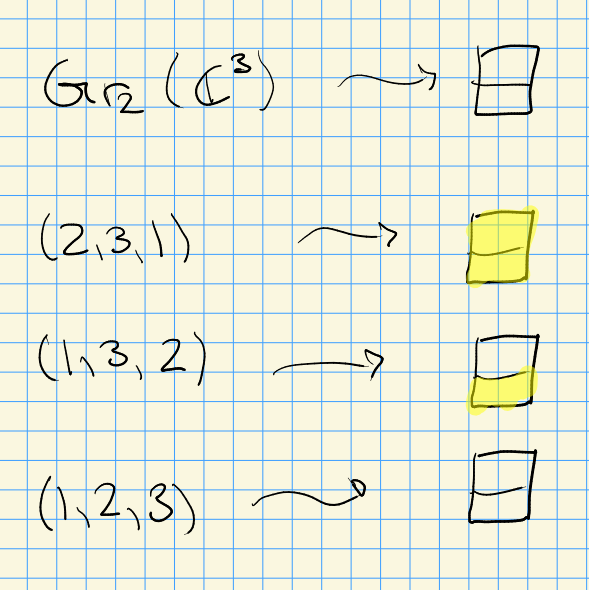• For $$Y = \left\{{1, 2}\right\}$$, we get $$W_Y = W$$ with a minimal element $$(1,2,3)$$.

Fix a reduced expression $$w = \prod_{i\leq n} s_i$$. Then $$v\leq w$$ iff there exist indices $$1\leq j_1 < j_2 < \cdots < j_p \leq n$$ such that $$v = \prod_{i\neq j_k} s_i$$.

For $$m_{12} = 3$$, if $$(s_1 s_2)^{m_{12} = 3} = e$$, so $$s_1 s_2 s_1 = s_2 s_1 s_2$$, which is a braid relation that corresponds to $$(3,2,1)$$. Let $$w_0$$ be the maximal element (which generally only works when the Coxeter group is finite), so here $$w_0 = s_1 s_2 s_1$$. We can cross out various reflections to get closure relations:Here for $$Y = \left\{{1}\right\} = \left\{{s_1}\right\}$$, we get minimal length elements $$e, s_2, s_1 s_2$$.

In general, we start with a GCM $$A$$, take a realization $$({\mathfrak{h}}, \pi, \pi {}^{ \vee })$$, get Kac-Moody Lie algebra $${\mathfrak{g}}$$, and extract a group $$W$$ which we now know is a Coxeter group. Write $$\left\{{{ {\alpha}_1, {\alpha}_2, \cdots, {\alpha}_{\ell}}}\right\} \subseteq {\mathfrak{h}} {}^{ \vee }$$ and $$S = \left\{{s_1, \cdots, s_\ell}\right\}$$, then for any $$1\leq i\leq \ell$$ set \begin{align*} s_i(\chi) \mathrel{\vcenter{:}}=\chi - {\left\langle {\chi},~{\alpha_i {}^{ \vee }} \right\rangle}\alpha_i && \forall \chi\in{\mathfrak{h}} {}^{ \vee } .\end{align*} Fix a real form $${\mathfrak{h}}_{\mathbb{R}}$$ of $${\mathfrak{h}}$$ satisfying

• $$\pi {}^{ \vee }\subseteq {\mathfrak{h}}_{\mathbb{R}}$$,
• $$\alpha_i({\mathfrak{h}}_{\mathbb{R}}) \subseteq {\mathbb{R}}$$ for all $$1\leq i \leq \ell$$.

Define the dominant chamber $$D_{\mathbb{R}}\subseteq {\mathfrak{h}}_{\mathbb{R}} {}^{ \vee }\mathrel{\vcenter{:}}=\mathop{\mathrm{Hom}}_{{\mathsf{{\mathbb{R}}}{\hbox{-}}\mathsf{Mod}}}({\mathfrak{h}}_{\mathbb{R}}, {\mathbb{R}})$$ as \begin{align*} D_{\mathbb{R}}\mathrel{\vcenter{:}}=\left\{{ \lambda\in {\mathfrak{h}}_{\mathbb{R}} {}^{ \vee }{~\mathrel{\Big\vert}~}\lambda(\alpha_i) \geq 0 \, \forall i}\right\} .\end{align*}

Define the Tits cone as \begin{align*} C \mathrel{\vcenter{:}}=\displaystyle\bigcup_{w\in W} wD_{\mathbb{R}} .\end{align*}

Consider the reductive group $${\mathsf{Sp}}_4({\mathbb{C}})$$, which is semisimple, simply connected, and connected. One way to realize this group is as \begin{align*} {\mathsf{Sp}}_4({\mathbb{C}}) \mathrel{\vcenter{:}}=\left\{{ g\in \operatorname{GL}_4({\mathbb{C}}) {~\mathrel{\Big\vert}~}\Theta(g) = g}\right\} \end{align*} for $$\Theta$$ some involution of $$\operatorname{GL}_4({\mathbb{C}})$$. Noting that we always have associated root datum $$(n, \left\{{ \alpha_i}\right\}_{i=1}^\ell ,\left\{{\alpha_i {}^{ \vee }}\right\}_{i=1}^\ell )$$, here we have \begin{align*} {\mathsf{Sp}}_4({\mathbb{C}}) = (2, \left\{{ (1,-1), (0, 2)}\right\}, \left\{{(1, -1), (0, 1)}\right\} ) .\end{align*} This yields a GCM \begin{align*} A = { \begin{bmatrix} {2} & {-2} \\ {-1} & {2} \end{bmatrix} } ,\end{align*} which comes from computing $$(A)_{ij} \mathrel{\vcenter{:}}=\alpha_i(\alpha_j {}^{ \vee })$$. Here \begin{align*} G/Z(G) = (2, \left\{{ (1, 0), (0, 1) }\right\} , \left\{{ (2, -2), (-1, 2) }\right\} ) .\end{align*} Note that these two root data are distinct over $${\mathbb{Z}}$$. We can consider the real form $${\mathfrak{h}}_{\mathbb{R}} {}^{ \vee }$$: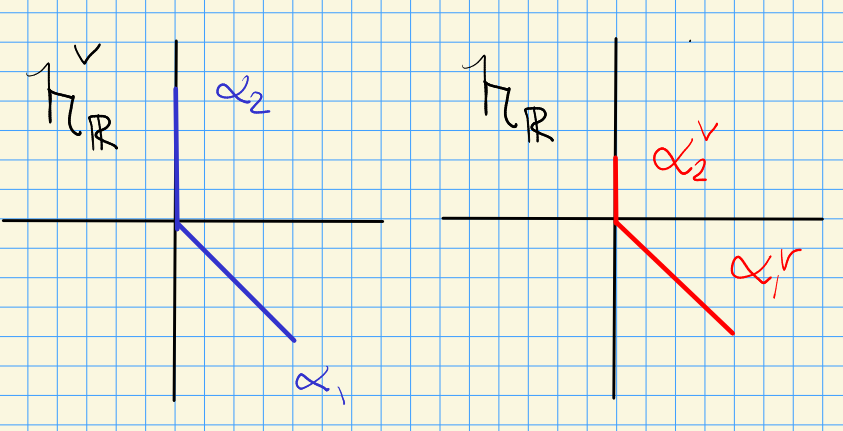We have

• $$\chi \in {\mathfrak{h}}_{\mathbb{R}} {}^{ \vee }= \left\{{(x, y)}\right\}$$,
• $$s_1(x, y) = (x, y) - {\left\langle {(x, y)},~{(1, -1)} \right\rangle}(1, -1) = (y, x)$$
• $$s_2(x, y) = (x, y) - {\left\langle {(x, y)},~{(0, 1)} \right\rangle}(0, 2) = (x, -y)$$

We can look at the $$W{\hbox{-}}$$orbits of these, and it turns out to recover all of the roots: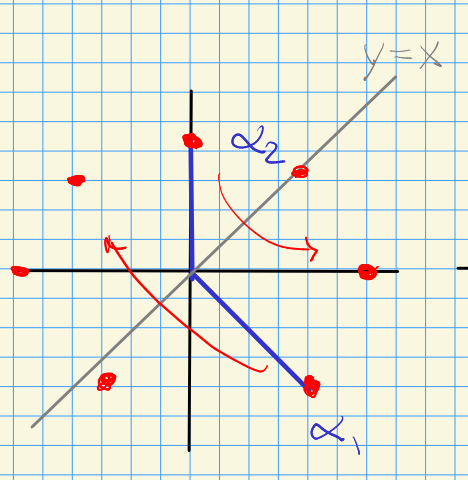$$W \subseteq \mathop{\mathrm{Aut}}({\mathfrak{h}}_{\mathbb{R}} {}^{ \vee })$$ is the subgroup given by $$\left\{{s_1, s_2}\right\}$$, and there are maps \begin{align*} s_1 s_2: (x, y) &\mapsto (-y, x)\\ s_2 s_1 s_2: (x, y) &\mapsto (y, -x)\\ s_1s_2 s_1 s_2: (x, y) &\mapsto (-x, -y)\\ s_2 s_1s_2 s_1 s_2: (x, y) &\mapsto (-x, y)\\ \vdots &\quad \vdots \\ (s_1s_2)^4: (x, y) &\mapsto (x, y) \implies m_{12} = 4 .\end{align*} Here we’ve used that $$(s_1 s_2)^2 = (s_2 s_1)^2$$. We can then find the dominant chamber: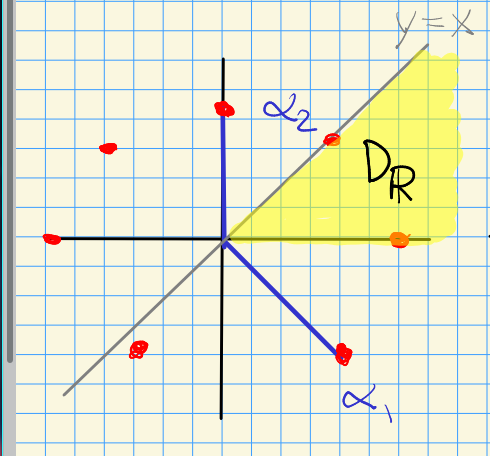For $$\lambda \in D_{\mathbb{R}}$$, we set $$W_\lambda \mathrel{\vcenter{:}}=\left\{{w\in W {~\mathrel{\Big\vert}~}w(\lambda) = \lambda}\right\}$$. This is generated by the simple reflections it contains. Setting $$Y = Y(\lambda) = \left\{{s_i \in S {~\mathrel{\Big\vert}~}\lambda( \alpha_i {}^{ \vee }) = 0}\right\}$$, we actually get $$W_\lambda = W_Y$$.

Recall what regular weights are!

# 9 Category $${\mathcal{O}}$$ (Friday, September 10)

Counterexamples: Kac Moodys that aren’t usual Lie algebras: affine Kac Moodys.

Our setup: $$A \leadsto ({\mathfrak{h}},\pi,\pi {}^{ \vee })$$. Fix $$\lambda \in {\mathfrak{h}} {}^{ \vee }$$ and $$c\in {\mathbb{C}}_{ \lambda} \ni z$$ a representation of $${\mathfrak{h}}$$ by $$x.\cdot \mathrel{\vcenter{:}}=\lambda(x)z$$. Recall that we have a triangular decomposition $${\mathfrak{g}}= {\mathfrak{n}}^- \oplus {\mathfrak{h}}\oplus {\mathfrak{n}}$$ with $${\mathfrak{h}}\oplus {\mathfrak{n}}\leq {\mathfrak{b}}$$ a subalgebra of the Borel. Since $${\mathfrak{n}}{~\trianglelefteq~}{\mathfrak{b}}$$ is an ideal, we can quotient to extend the representation \begin{align*} {\mathfrak{b}}\to {\mathfrak{b}}/{\mathfrak{n}}\cong {\mathfrak{h}}\xrightarrow{\lambda} {\mathbb{C}}_{\lambda} .\end{align*} This extends from $${\mathfrak{h}}$$ to $${\mathfrak{b}}$$ by making it zero on $${\mathfrak{n}}$$, and generally one can do this with nilradicals.

\begin{align*} M(\lambda) \mathrel{\vcenter{:}}= U({\mathfrak{g}}) \otimes_{U({\mathfrak{b}})} {\mathbb{C}}_{\lambda} \in {\mathsf{{\mathfrak{g}}}{\hbox{-}}\mathsf{Mod}} ,\end{align*} where $${\mathfrak{b}}\curvearrowright{\mathbb{C}}_{\lambda}$$ extends to the universal enveloping algebra.

The PBW theorem implies that every $$M(\lambda)\cong U({\mathfrak{n}}^-) \otimes_{\mathbb{C}}{\mathbb{C}}_{\lambda}$$ as vector spaces, which is in fact an isomorphism in $${\mathsf{{\mathfrak{b}}^-}{\hbox{-}}\mathsf{Mod}}$$. This means $$M( \lambda)$$ is a weight module for $${\mathfrak{h}}$$, i.e. there is a decomposition $$M(\lambda) = \bigoplus_{\mu \in {\mathfrak{h}} {}^{ \vee }}M(\lambda)_{ \mu}$$ where \begin{align*} M(\lambda)_{\mu} \mathrel{\vcenter{:}}=\left\{{v\in M( \lambda) {~\mathrel{\Big\vert}~}h\cdot v = \mu(h) v,\,\quad h\in {\mathfrak{h}}}\right\} .\end{align*}

Any nonzero quotient $$L$$ of $$M(\lambda)$$ in $${\mathsf{{\mathfrak{g}}}{\hbox{-}}\mathsf{Mod}}$$ is a highest weight module with highest weight $$\lambda$$.

Why highest weight? There is a partial order on weights: \begin{align*} \mu \leq \lambda\iff \lambda- \mu\in Q^+ \mathrel{\vcenter{:}}={\mathbb{Z}}_{> 0} \pi .\end{align*} Also note that $$M( \lambda)$$ is a highest weight module.

There is a full subcategory $${\mathcal{O}}\leq {\mathsf{{\mathfrak{g}}}{\hbox{-}}\mathsf{Mod}}$$ where every $$M\in {\operatorname{Ob}}({\mathcal{O}})$$ satisfies the following:

• (Finite multiplicities) $$M$$ is a weight module with finite-dimensional weight spaces.
• There exist finitely many weights $${ {\lambda}_1, {\lambda}_2, \cdots, {\lambda}_{k}}\in {\mathfrak{h}} {}^{ \vee }$$ (depending on $$M$$) such that $$P(M) \subseteq \displaystyle\bigcup_{1\leq j\leq k} {\mathfrak{h}} {}^{ \vee }_{\leq \lambda_j}$$: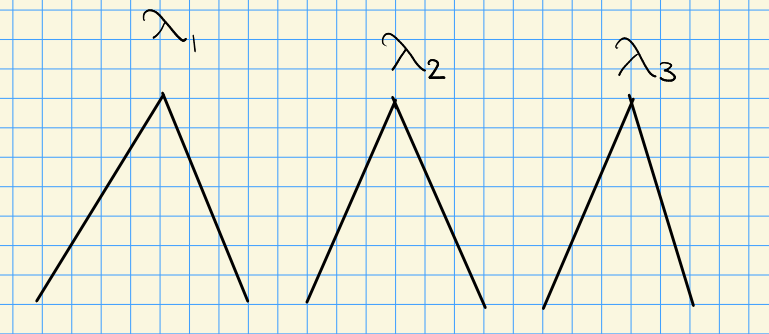Any $$M(\lambda)$$ has a unique proper maximal $${\mathfrak{g}}{\hbox{-}}$$submodule $$M'(\lambda)$$. In particular, $$\lambda\not \in M'(\lambda)$$, and there is a unique irreducible quotient $$L(\lambda) \mathrel{\vcenter{:}}= M( \lambda)/M'( \lambda)$$.

The proof is easy: use that $$\lambda$$ generated $$M(\lambda)$$ as a $${\mathfrak{g}}{\hbox{-}}$$module.

For any irreducible $$L \in {\operatorname{Ob}}({\mathcal{O}})$$, there exists a unique $$\lambda \in {\mathfrak{h}} {}^{ \vee }$$ such that $$L \cong L(\lambda)$$.

Define the dominant integral weights \begin{align*} D \mathrel{\vcenter{:}}=\left\{{ \lambda\in {\mathfrak{h}} {}^{ \vee }{~\mathrel{\Big\vert}~}\forall \alpha_i {}^{ \vee }\in \pi {}^{ \vee },\,\, {\left\langle { \lambda},~{\alpha_i {}^{ \vee }} \right\rangle} \in {\mathbb{Z}}_{> 0} }\right\} .\end{align*}

For $$\lambda \in D$$, define $$M_1(\lambda) \subseteq M( \lambda)$$ as the submodule generated by $$\left\{{ f_i^{ \lambda(\alpha_i {}^{ \vee }) + 1 } \otimes 1}\right\}_{i=1}^\ell$$, and define \begin{align*} L^{\max}( \lambda) \mathrel{\vcenter{:}}={M(\lambda) \over M_1( \lambda)} ,\end{align*} the operators that act locally nilpotently (so there is an exponent depending on the vector)

Let $$A = {\left[ {2} \right]}$$ be a $$1\times 1$$ GCM, which yields $$({\mathbb{C}}, \left\{{ 2}\right\}, \left\{{ 1}\right\})\leadsto {\mathfrak{sl}}_2({\mathbb{C}})$$. Given $$\lambda\in {\mathbb{C}},$$ we have \begin{align*} M( \lambda) &= U({\mathfrak{sl}}_2) \otimes_{U({\mathfrak{b}})} {\mathbb{C}}_{\lambda} \\ &\cong U({\mathfrak{n}}^-) \otimes_{\mathbb{C}}{\mathbb{C}}_{\lambda} \\ &= {\mathbb{C}}[y] \otimes_{\mathbb{C}}{\mathbb{C}}_{ \lambda} .\end{align*} where noting that $${\mathfrak{n}}^- = \left\langle{f_i}\right\rangle$$ and $${\mathfrak{n}}= \left\langle{e_i}\right\rangle$$, we identify the variable $$y$$ with $$f$$.

This has weights $$\lambda, \lambda-2, \lambda-4, \cdots$$, identifying elements as $$y^k \otimes 1$$. How do $$e,f,h \in {\mathfrak{g}}$$ act in this basis?

• $$h(y^k \otimes 1) = (hy^k) \otimes 1 = (\lambda - 2k)(y^k\otimes 1)$$.
• $$f(y^k\otimes 1) = y(y^k\otimes 1) = y^{k+1}\otimes 1$$.
• $$e$$: more complicated!

The game: move $$e$$s across the tensor product to kill terms:

• For $$k=0$$: \begin{align*} e(1\otimes 1) = e\otimes 1 = 1\otimes e(1) = 0 \end{align*} since we extended $$\lambda$$ by zero on $${\mathfrak{n}}$$.

• For $$k=1$$: \begin{align*} e(y\otimes 1) &= e(f\otimes 1) \\ &= (ef)\otimes 1 \\ &= ([ef] + fe)\otimes 1 \\ &= [ef] \otimes 1 \\ &= \alpha {}^{ \vee }\otimes 1 \\ &= 1\otimes\alpha {}^{ \vee }\cdot 1 \\ &= \lambda( \alpha {}^{ \vee })(1\otimes 1) \\ &= \lambda ,\end{align*} using $$ef = [e,f] + fe = ef-fe + fe$$ and $$fe\otimes 1 = f\otimes e(1) = 0$$.

• For $$k=2$$: \begin{align*} eff\otimes 1 &= ([ef] + fe)f \otimes 1 \\ &=( \alpha {}^{ \vee }f + fef )\otimes 1 \\ &= ( \alpha {}^{ \vee }f + f([ef] + fe) ) \otimes 1 \\ &= (\alpha {}^{ \vee }f + f[ef]) \otimes 1 \\ &= (\alpha {}^{ \vee }f + f \alpha {}^{ \vee }) \otimes 1 && f \alpha {}^{ \vee }\in {\mathfrak{h}}\\ &= ( \alpha {}^{ \vee }f + \lambda f) \otimes 1 \\ &= ( [\alpha {}^{ \vee }, f] + f \alpha {}^{ \vee }+ \lambda f) \otimes 1 \\ &= ( - \alpha( \alpha {}^{ \vee }) f + 2 \lambda f) \otimes 1 && \text{using Kac-Moody relns.} \\ &= 2( \lambda- 1)f \otimes 1 .\end{align*}

Then general pattern is $$e(y^k \otimes 1) = k ( \lambda - (k-1) ) \qty{ y^{k-1} \otimes 1 }$$.

Here \begin{align*} D =\left\{{ \lambda\in {\mathfrak{h}} {}^{ \vee }= {\mathbb{C}}{~\mathrel{\Big\vert}~}{\left\langle { \lambda},~{ \alpha {}^{ \vee }} \right\rangle} \in {\mathbb{Z}}_{>0} }\right\} = {\mathbb{Z}}_{>0} \subseteq {\mathbb{C}}= {\mathfrak{h}} {}^{ \vee } \end{align*} and for $$\lambda \in D$$,
\begin{align*} M_1( \lambda) = \left\{{f^{ \lambda( \alpha_i {}^{ \vee }) + 1} \otimes 1}\right\}_{1\leq i \leq \ell = 1} = \left\{{f^{\lambda+ 1} \otimes 1}\right\} .\end{align*} Note that $$e\cdot f^{ \lambda+1}\otimes 1 = 0$$, which can be checked from the above formula: \begin{align*} e(y^{\lambda+1} \otimes 1) = ( \lambda+1)( \lambda- \lambda) y^{ \lambda} = 0 .\end{align*} Thus $$M_1( \lambda) = {\mathbb{C}}\left\langle{y^{\lambda+1}, y^{ \lambda+2}, \cdots}\right\rangle$$. Finally, \begin{align*} {M( \lambda) \over M_1( \lambda)} = L^{\max}( \lambda) = L( \lambda) .\end{align*}

# 10 Tits Systems, 5.1 (Monday, September 13)

The basic setup from the book: \begin{align*} A\leadsto ({\mathfrak{h}}, \pi, \pi {}^{ \vee }) \leadsto {\mathfrak{g}}\leadsto (W, S) .\end{align*} We’ll think of $$G\leadsto ({\mathfrak{h}}, \pi, \pi {}^{ \vee })$$ as the root data associated to a semisimple simply connected connected algebraic group. Warning: this association isn’t unique in the non-semisimple case! Noting that $$(W, S)$$ is a Coxeter group, is there a way to recover an algebra $${\mathfrak{g}}$$ and a Kac-Moody group $${\mathcal{G}}$$?

For today: take

• $$G\mathrel{\vcenter{:}}=\operatorname{GL}_n$$, Note that $$G$$ is not semisimple or simply connected.

• $$B$$ the fixed Borel (maximum connected closed solvable subgroup) of upper-triangular matrices. Flag varieties are homogeneous projective spaces, so $$G/B$$ is a flag variety.

• $$T$$ the maximal torus of diagonal matrices

• $$N = N_G(T)$$ to be the subgroup generated by all permutation and scalar matrices.

• The Weyl group $$W\mathrel{\vcenter{:}}= N/ B \cap N = N/T$$ since $$B \cap N = T$$. Note that $$W\cong S_n$$ is a Coxeter group.

• $$S \subseteq W$$ is the subset of simple reflections, writing $$w = (w_1, \cdots, w_n)$$ and taking only those permutations that transpose two adjacent coordinates, so \begin{align*} \tau_{k}: (w_1, \cdots, w_k, w_{k+1}, \cdots, w_n) &\mapsto (w_1, \cdots, w_{k+1}, w_{k}, \cdots, w_n) .\end{align*}

This can be written as $$\left\langle{\tau_k}\right\rangle \mathrel{\vcenter{:}}=\left\langle{(k, k+1) {~\mathrel{\Big\vert}~}{1\leq k \leq n-1}}\right\rangle$$.

More generally, $$G \supseteq B \supseteq T$$ and we set $$W \mathrel{\vcenter{:}}= N_G(T) / Z_G(T)$$ and show $$Z_G(T) = T$$, but what is $$B \cap N$$ generally? Maybe use the fact that $$N_G(B) = B$$? Or that the unipotent radical intersects it trivially.

A Tits system is a tuple $$(G,B,N,S)$$ where $$B,N\leq G$$ are subgroups and $$S \subseteq W = N/B \cap N$$, which collectively adhere to the following axioms:

1. $$B \cap N {~\trianglelefteq~}N$$,
2. $$B, N$$ generate $$G$$,
3. For all $$s_i\in S$$, we have $$sBs^{-1}\not\subseteq B$$
4. For $$w\in S_n$$ and $$s\in S$$, defining $$C(x) \mathrel{\vcenter{:}}= B \mkern 1.5mu\overline{\mkern-1.5mux\mkern-1.5mu}\mkern 1.5mu B \subseteq G$$ for any coset representative $$\mkern 1.5mu\overline{\mkern-1.5mux\mkern-1.5mu}\mkern 1.5mu$$ of $$x$$ in $$N$$, we require $$C(s) C(w) \subseteq C(w) \cup C(sw)$$.

Consider elements in $$BN$$ for $$\operatorname{GL}_n$$: $$B$$ is upper triangular, $$N$$ has one (possibly) nonzero entry in each row/column, and multiplying this can “smear” the entries upward by filling a column above an entry: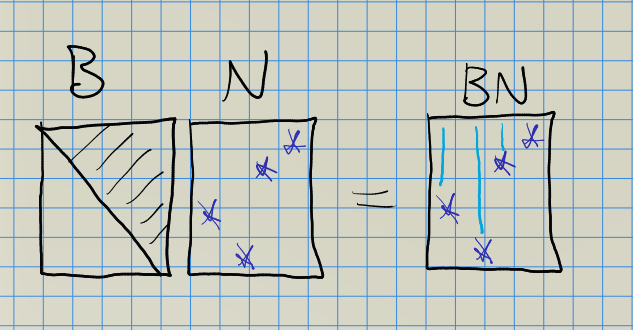Similarly, multiplying on the right smears rightward, and it’s not so hard to convince yourself that these generate $$\operatorname{GL}_n$$.

For the conjugation axiom, consider the following: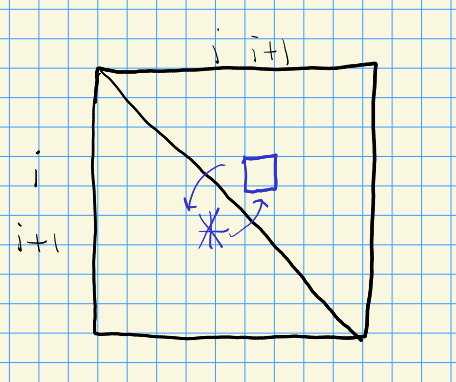We also have $$B\mkern 1.5mu\overline{\mkern-1.5mus\mkern-1.5mu}\mkern 1.5mu B\mkern 1.5mu\overline{\mkern-1.5muw\mkern-1.5mu}\mkern 1.5mu B \subseteq B\mkern 1.5mu\overline{\mkern-1.5muw\mkern-1.5mu}\mkern 1.5mu B \cup B \mkern 1.5mu\overline{\mkern-1.5mus\mkern-1.5mu}\mkern 1.5mu \mkern 1.5mu\overline{\mkern-1.5muw\mkern-1.5mu}\mkern 1.5mu B$$. To prove this, we’ll show

• $$\mkern 1.5mu\overline{\mkern-1.5mus\mkern-1.5mu}\mkern 1.5mu B \mkern 1.5mu\overline{\mkern-1.5muw\mkern-1.5mu}\mkern 1.5mu \subseteq$$ the RHS,
• The right-hand side is stable under the $$B\times B$$ action of left/right multiplication.

To see the first, consider the example: